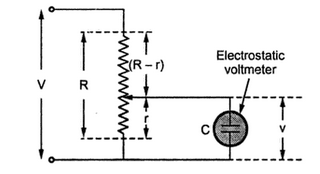### Extension of Range of Electrostatic Instruments

The range of various instruments can be extended using multipliers. Similarly the range of electrostatic instruments is also extended using multipliers. The multipliers used for electrostatic instruments are of two types,
1.Resistance potential divider   2.Capacitance multipliers
1.1 Resistance Potential  Divider
The use of resistance potential divider for extending the range of an electrostatic instrument is shown in the Fig 1.Fig. 1 Use of resistance potential divider

R = Total resistance of potential divider
V = Voltage to be measured
r = The resistance whose voltage drop is applied to an electrostatic voltmeter
v= The voltage across an electrostatic voltmeter
C= The capacitance of an electrostatic voltmeter
The resistance r and capacitance C forms a parallel circuit and the equivalent impedance is,

Thus the equivalent impedance across the voltage V is,

The factor by which voltage is changed due to potential divider is called its multiplying power and given by,

The numerical value of multiplying power m is,

If ω, C and r are very small then ω2 C2 r2 ≤1 and can be neglected,

1.At high voltages, the cost of this method is high.
2.At high voltages, the power loss and wastage is excessive.
3.At high voltages, the accuracy is very less due to stray capacitance effects.
4.When used for a.c. measurements, it should be wound noninductively and capacitor leakage resistance must be high.
Thus this method is not suitable for high voltages but used for d.c. measurements as capacitance potential divider can not be used for d.c. circuits.
1.2 Capacitance Multipliers
The capacitance multiplier method is nothing but the use of capacitance potential divider. There are two methods of connecting capacitor for potential division.
Method 1: In first method, a single capacitor is connected in series with the voltmeter and the voltage to be measured is applied across the combination as shown in the Fig 2.Fig. 2 Capacitor multiplier method

Let         C = Series capacitor
Cv = Capacitor of voltmeter
v = Voltage across voltmeter
V = Voltage to be measured
The total capacitance across the supply is,

The total impedance across the supply is,

The impedance of voltmeter is,

Thus the multiplying power of the multiplier is,

1. To have high value of multiplying power, the voltmeter capacitor must be high.
2. The voltmeter capacitor varies with the deflection of the moving needle hence te voltmeter must be calibrated alongwith the series multiplier capacitor.
Method 2: In many practical cases a set of capacitors connected in series across the voltage be measured as shown in the Fig 3.Fig.2 Capacitor multiplier - method 2

The capacitors C1 and Cv are in parallel hence their resultant is C1+ Cv. While C2, C3Cn are in series and their equivalent is Cs   where,

Thus Cs and (C1+ Cv ) are in series hence the resultant capacitor across the voltage V is,

While across the voltage v the capacitor is C1+ Cv hence the impedance is,

Thus the multiplying power is,

If C1 is large with respect to Cv, then there is no appreciable change in the multiplying power alongwith the deflection of the pointer.

1.2.